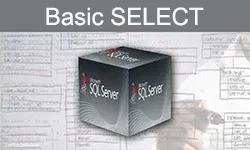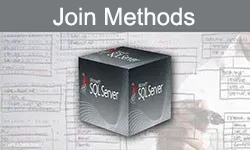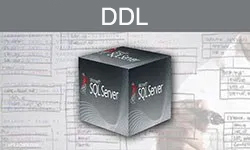Select PageThis tutorial illustrates the core concepts of the SQL language in a logical and sorted manner. This SQL tutorial covers a broad range of topics, starting from basic queries and filtering data, sorting a result set, querying multiple tables, using various functions and creating new database structures

In order to help you better understand different concepts, most of these tutorials are accompanied by exercises (including solutions), allowing you to practice the SQL Language. To download the databases used in these exercises, use one of the following links: [ACDB] [HR].Basic Select Statements

Exercises ACDB | Exercises HR

This tutorial explains how  to write basic SELECT statements, how  to use arithmetic operators on different values, how to change column headings using column aliases, and how to concatenate two or more string values. In this tutorial you will also learn how to return only distinct (different) values using the DISTINCT statement.Filtering and Sorting the results

Exercises ACDB | Exercises HR

This tutorial explains how to use the WHERE clause and how to filter the results using comparison operators (=, <, >, =>, etc.) and logical operators (IN, BETWEEN, LIKE, IS NULL, AND & OR).

This tutorial also explains how to sort the results using the ORDER BY clause and how to specify the number of rows to return using the TOP statement.Scalar Functions

Exercises ACDB | Exercises HR

Scalar Functions are used to carry out operations such as: mathematical tasks on numerical data, different manipulations on string and dates values, NULL-related operations, and datatypes conversions. This guide provides you a summary of some of the most common SQL Server Built-in Scalar Functions.Group Functions

Exercises ACDB | Exercises HR

This tutorial explains how to use group functions, how to summarize your data using the GROUP BY clause, and how to restrict the groups of returned rows using the HAVING clause.Join Methods

Exercises ACDB | Exercises HR

This tutorial explains how to combine rows from two or more tables using INNER JOIN and OUTER JOIN. This tutorial also explains how to use SELF JOIN in order to join a table to itself.Sub Queries

Exercises ACDB | Exercises HR

This tutorial explains how to nest a query within another SQL query. This tutorial also explains the difference between Single-row and multiple-row subqueries.Add, Modify, or Remove Data from a Table

This tutorial explains how to insert new rows into a table using the INSERT statement, how to update existing fields using the UPDATE statement and how to remove existing rows using the DELETE statement.DDL

This tutorial explains how to create new tables using the CREATE TABLE statement, how to modify the table structure using the ALTER TABLE statement and how to delete existing tables using the DROP TABLE statement.